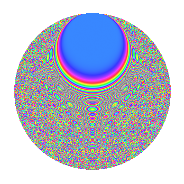# Properties

 Label 525.4.bbLevel $525$ Weight $4$ Character orbit 525.bb Rep. character $\chi_{525}(41,\cdot)$ Character field $\Q(\zeta_{10})$ Dimension $944$ Sturm bound $320$

# Related objects

## Defining parameters

 Level: $$N$$ $$=$$ $$525 = 3 \cdot 5^{2} \cdot 7$$ Weight: $$k$$ $$=$$ $$4$$ Character orbit: $$[\chi]$$ $$=$$ 525.bb (of order $$10$$ and degree $$4$$) Character conductor: $$\operatorname{cond}(\chi)$$ $$=$$ $$525$$ Character field: $$\Q(\zeta_{10})$$ Sturm bound: $$320$$

## Dimensions

The following table gives the dimensions of various subspaces of $$M_{4}(525, [\chi])$$.

Total New Old
Modular forms 976 976 0
Cusp forms 944 944 0
Eisenstein series 32 32 0

## Trace form

 $$944q + 916q^{4} - 28q^{7} - 6q^{9} + O(q^{10})$$ $$944q + 916q^{4} - 28q^{7} - 6q^{9} - 46q^{15} - 3660q^{16} - 64q^{18} + 102q^{21} - 196q^{22} + 272q^{25} - 206q^{28} - 86q^{30} + 628q^{36} + 204q^{37} + 898q^{39} + 1027q^{42} - 1376q^{43} - 1564q^{46} + 980q^{49} + 1268q^{51} + 1596q^{57} - 6496q^{58} + 544q^{60} - 2158q^{63} + 14844q^{64} + 2004q^{67} + 3636q^{70} - 4218q^{72} + 6212q^{78} - 1188q^{79} + 2470q^{81} - 150q^{84} + 3532q^{85} - 9168q^{88} + 1008q^{91} + 2884q^{93} - 4116q^{99} + O(q^{100})$$

## Decomposition of $$S_{4}^{\mathrm{new}}(525, [\chi])$$ into newform subspaces

The newforms in this space have not yet been added to the LMFDB.

## Hecke characteristic polynomials

There are no characteristic polynomials of Hecke operators in the database